﻿ Row reduction with the TI83 or TI84 calculator (rref) - MathBootCamps

# Row reduction with the TI83 or TI84 calculator (rref)

Row reducing a matrix can help us find the solution to a system of equations (in the case of augmented matrices), understand the properties of a set of vectors, and more. Knowing how to use row operations to reduce a matrix by hand is important, but in many cases, we simply need to know what the reduced matrix looks like. In these cases, technology like a graphing calculator is a great tool to use!

We will go through the steps using this matrix: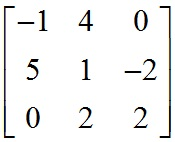## Step 1: Go to the matrix menu on your calculator.

Press [2nd][x^-1] to enter the matrix menu. Note that some older calculators have a button that simply says [MATRX]. Press the right arrow until you are under the EDIT menu.Press [ENTER] and you can now edit matrix A.

## Step 2: Enter your matrix into the calculator.

The first information you are asked is the size of the matrix. This matrix has 3 rows and 3 columns, so it is a 3 x 3 matrix. Type these numbers, pressing [ENTER] after each.Now you can enter each number by typing it and pressing [ENTER].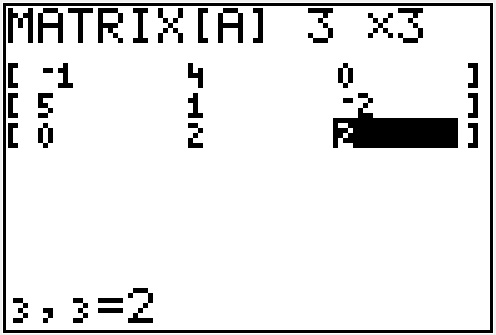## Step 3: Quit out of the matrix editing screen.

This is a strange step, but if we don’t do it, the calculator tries to put a reduced matrix INSIDE of this matrix. It’s a bug for sure, but one we can work around.

Press [2nd] and then [MODE] to quit. You will end up at a blank screen.

## Step 4: Go to the matrix math menu.

Press [2nd][X^-1] to enter the matrix menu again, but this time go over to MATH.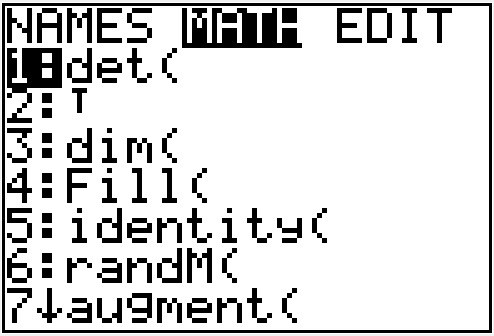Scroll down to “rref” (reduced row echelon form) and press [ENTER].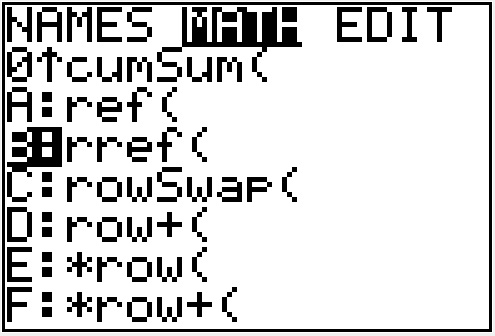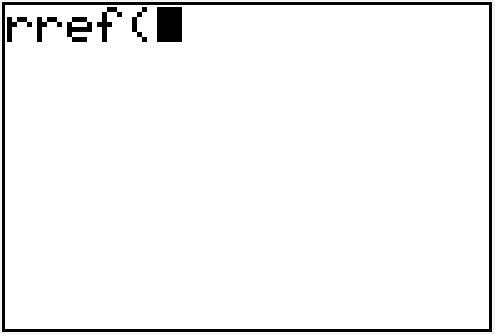## Step 5: Select matrix A and finally row reduce!

To select matrix A, you need to go back into the matrix menu by pressing [2nd][x^-1] but stay under the NAMES menu.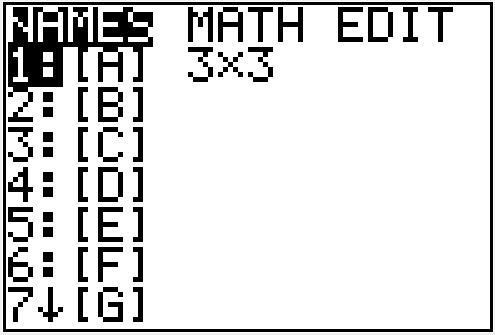Now press [ENTER] to select matrix A.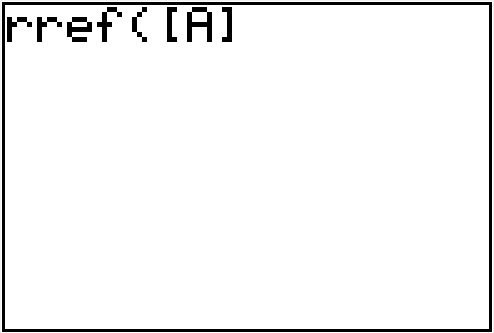Close your parentheses by pressing [ ) ] and then pressing [ENTER] to get the reduced matrix.

(note: you don’t have to close the parentheses for this to work, but it is a good habit – or maybe just not closing parentheses drives me crazy… one of those..).Interesting! This matrix turns out to be row equivalent to the identity matrix. If you are currently studying linear algebra, you know that this is a useful fact to know about a matrix. There are many properties that are now automatically true for our matrix A!

Either way, we are done. We now have row reduced matrix A.

## Fractions vs. Decimals

One thing that can happen when you row reduce, is that you end up with messy decimals. With one additional step, you can convert these to fractions. So, suppose we reduced a matrix and ended up with the following: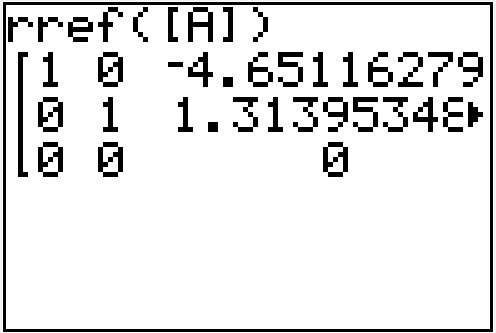Before pressing any other buttons, press [MATH] and then select >FRAC by pressing [ENTER].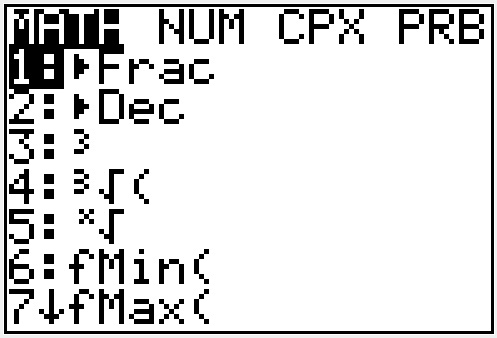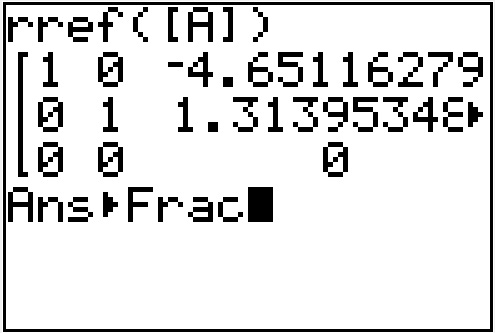Now press [ENTER] to get a reduced matrix with fractions instead of decimals.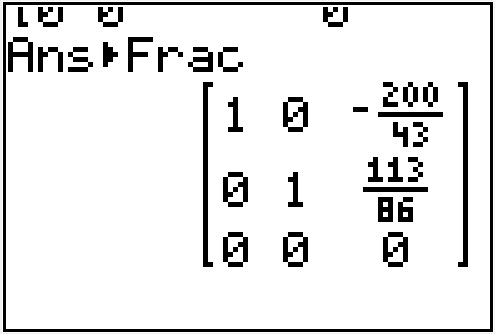This is much better! You will want to use this for most mathematical problems, as the fractions are exact values and the decimals before were approximations.